In DAX, variables should be used extensively as a way to improve the readability of an expression. However, using variables properly is also helpful for query performance. Indeed,  this suggests a better execution plan in case the same variable or sub-expression is referenced more than once in the same filter context.

For example, consider this simple implementation of the Dynamic Segmentation pattern in a Power BI data model.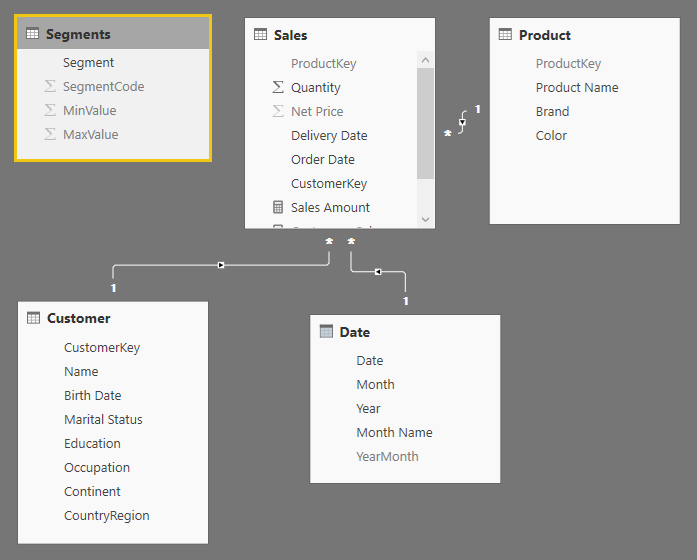The Segments table defines ranges for three segments that will be used to cluster customers based on their spending.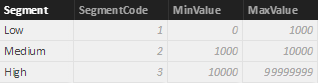The original Dynamic Segmentation pattern was written before variables were available. Thus, the first implementation of the segmented Customer Sales measure is the following:

```Sales[Customers Sales] :=
IF (
ISCROSSFILTERED ( Segments ),
SUMX (
Segments,
SUMX (
Customer,
IF (
[Sales Amount] >= Segments[MinValue]
&& [Sales Amount] < Segments[MaxValue],
[Sales Amount]
)
)
),
[Sales Amount]
)
```

The report obtained by using the Customer Sales measure shows appropriate results.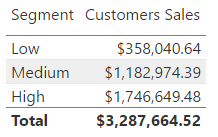However, there are three references to the Sales Amount measure – within the same filter and row contexts – in the SUMX iteration of the Customer table. In an ideal world, the query engine should be able to identify that the result of the three references will always be the same for a given customer. This could be true in the future, but this is not the case as of January 2018.

Using DAX Studio, it is possible to see the storage engine queries generated by the Customer Sales measure in the previous report.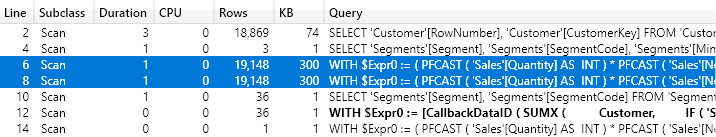The two highlighted lines show a similar datacache returned by similar storage engine queries. Both have the same number of rows and the same size, and the initial part of the query looks identical. Nevertheless, there are differences in the two storage engine queries. The first engine query is the following.

```WITH
\$Expr0 := ( PFCAST ( 'Sales'[Quantity] AS  INT )
* PFCAST ( 'Sales'[Net Price] AS  INT )  )
SELECT
'Customer'[CustomerKey],
SUM ( @\$Expr0 )
FROM 'Sales'
LEFT OUTER JOIN 'Customer' ON
'Sales'[CustomerKey]='Customer'[CustomerKey];
```

The second engine query presents an additional filter condition.

```WITH
\$Expr0 := ( PFCAST ( 'Sales'[Quantity] AS  INT )
* PFCAST ( 'Sales'[Net Price] AS  INT )  )
SELECT
'Customer'[CustomerKey],
SUM ( @\$Expr0 )
FROM 'Sales'
LEFT OUTER JOIN 'Customer' ON
'Sales'[CustomerKey]='Customer'[CustomerKey]
WHERE
'Customer'[CustomerKey] IN (
13407, 9266, 17548, 7787, 3646, 17055, 12914, 18041, 7294,
3153..[18869 total values, not all displayed]
) ;
```

Remember that the storage engine query shows an estimated row size for each datacache. The real number is available in the Spool_Iterator event of the physical query plan and it corresponds to 18,869, which is the actual number of customers.

The result of both datacaches is identical. There are two requests made to the storage engine for the same result, because the query optimizer assumes possible different results for the two arguments of the IF function. A deeper analysis of the query semantic should allow the query optimizer to do a better job, but this is not happening as of January 2018. Using variables, it is possible to evaluate the Sales Amount measure only once for each customer, referencing the variable value in the different arguments of the IF function.

The following is the optimized version of the Customer Sales measure.

```Sales[Customers Sales Optimized] :=
IF (
ISCROSSFILTERED ( Segments ),
SUMX (
Segments,
SUMX (
Customer,
VAR SalesAmount = [Sales Amount]
RETURN
IF (
SalesAmount >= Segments[MinValue]
&& SalesAmount < Segments[MaxValue],
SalesAmount
)
)
),
[Sales Amount]
)
```

Using this measure, the physical query plan is shorter and there is a single datacache materializing the result of Sales Amount for every customer.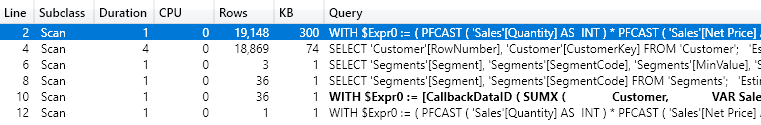The highlighted line is the single datacache used to compute Sales Amount for all the customers, and it does not contain any filter condition.

```WITH
\$Expr0 := ( PFCAST ( 'Sales'[Quantity] AS  INT )
* PFCAST ( 'Sales'[Net Price] AS  INT )  )
SELECT
'Customer'[CustomerKey],
SUM ( @\$Expr0 )
FROM 'Sales'
LEFT OUTER JOIN 'Customer' ON
'Sales'[CustomerKey]='Customer'[CustomerKey];
```

The smaller materialization improves query performance. This is barely measurable in the small data models available as a download, but the difference is visible to the user in data models with a large number of customers.

The same performance optimization is possible when the same subexpression is evaluated multiple times in the same evaluation context. For example, this is a version of Customer Sales that does not include measure references and repeats the same CALCULATE multiple times instead.

```Sales[Customers Sales exp] :=
IF (
ISCROSSFILTERED ( Segments ),
SUMX (
Segments,
SUMX (
Customer,
IF (
CALCULATE ( SUMX ( Sales, Sales[Quantity] * Sales[Net Price] ) )
>= Segments[MinValue]
&& CALCULATE ( SUMX ( Sales, Sales[Quantity] * Sales[Net Price] ) )
< Segments[MaxValue],
CALCULATE ( SUMX ( Sales, Sales[Quantity] * Sales[Net Price] ) )
)
)
),
[Sales Amount]
)
```

The optimized version presents the same approach shown previously. There is a single evaluation of the CALCULATE statement, and its result is reused multiple times referencing the SalesAmount variable.

```Sales[Customers Sales exp Optimized] :=
IF (
ISCROSSFILTERED ( Segments ),
SUMX (
Segments,
SUMX (
Customer,
VAR SalesAmount = CALCULATE ( SUMX ( Sales, Sales[Quantity] * Sales[Net Price] ) )
RETURN
IF (
SalesAmount >= Segments[MinValue]
&& SalesAmount < Segments[MaxValue],
SalesAmount
)
)
),
[Sales Amount]
)
```

In DAX, variables provide better code readability and facilitate the generation of a more optimized query plan by reducing the chances of computing the same sub-expression multiple times.

SUMXReturns the sum of an expression evaluated for each row in a table.

`SUMX ( <Table>, <Expression> )`

IFChecks whether a condition is met, and returns one value if TRUE, and another value if FALSE.

`IF ( <LogicalTest>, <ResultIfTrue> [, <ResultIfFalse>] )`

CALCULATEContext transition

Evaluates an expression in a context modified by filters.

`CALCULATE ( <Expression> [, <Filter> [, <Filter> [, … ] ] ] )`